# Surface Area and Volume Class 9

According to the CBSE Syllabus 2023-24, this chapter has been renumbered as Chapter 11.

## CBSE Class 9 Maths Surface Areas and Volumes Notes:-

The surface area and volume for Class 9 notes are given here. In this article, the complete surface area and volume formulas for different three-dimensional shapes are discussed with a complete explanation. For any three-dimensional figures, the surface area can be broadly classified into Curved Surface Area(CSA), Lateral Surface Area (LSA), and Total Surface Area (TSA). These can be computed for 3D shapes, such as a cube, a cuboid, a cone, a cylinder and so on.

## Class 9 Maths Chapter 13 Surface Areas and Volumes

Students will be introduced to the surface areas and volumes for different shapes such as cuboid, cube, right circular cylinder, right circular cone and sphere. Let us see the important notes and formulas for each of the shapes.

### Cuboid

A cuboid is a three-dimensional Shape. The cuboid is made of six rectangular faces, which are placed at right angles. The total surface area of a cuboid is equal to the sum of the areas of its six rectangular faces.

### Total Surface Area of a Cuboid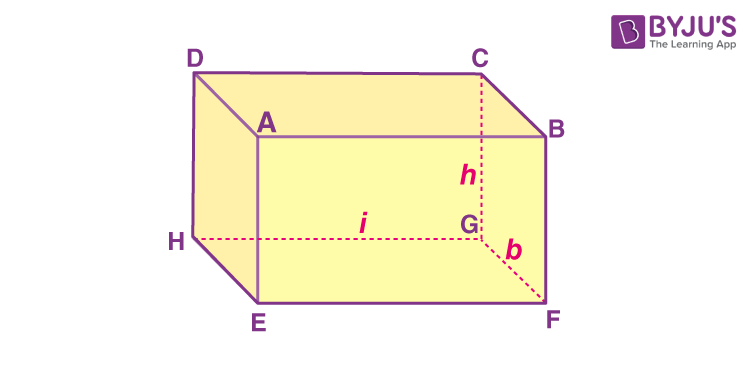Consider a cuboid whose length is “l” cm, breadth is b cm and height h cm.
Area of face ABCD = Area of Face EFGH (× bcm2
Area of face AEHD = Area of face BFGC (× hcm2
Area of face ABFE = Area of face DHGC (× hcm2
Total surface area (TSA) of cuboid = Sum of the areas of all its six faces
2(× b2(× h2(× h)
TSA (cuboid)= 2(lb + bh +lh)

### Lateral Surface Area of a Cuboid

Lateral surface area (LSA) is the area of all the sides apart from the top and bottom faces.
The lateral surface area of the cuboid
= Area of face AEHD + Area of face BFGC + Area of face ABFE + Area of face DHGC
2(× h2(× h)
LSA (cuboid) = 2h(l + b)

### Cube

A cuboid whose length, breadth and height are all equal is called a cube. It is a three-dimensional shape bounded by six equal squares. It has 12 edges and 8 vertices.

### Total Surface Area of a Cube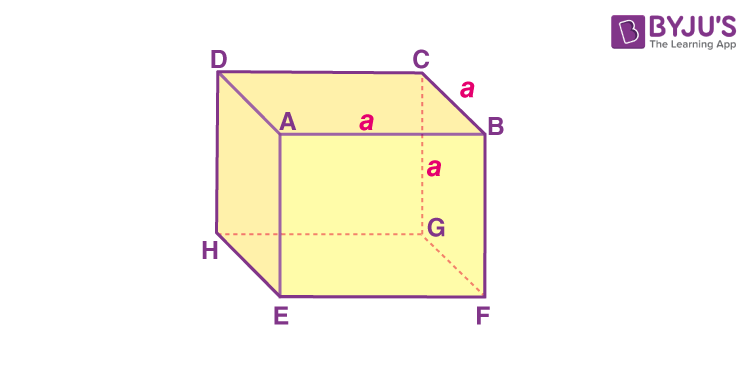For cube, length = breadth = height
Suppose the length of an edge = a
Total surface area(TSA) of the cube 2(× × × a)
TSA(cube) × (3a26a2

### Lateral Surface Area of a Cube

Lateral surface area (LSA) is the area of all the sides apart from the top and bottom faces.
Lateral surface area of cube = 2(× × a4a2

#### For more information on Cube And Cuboids, watch the below video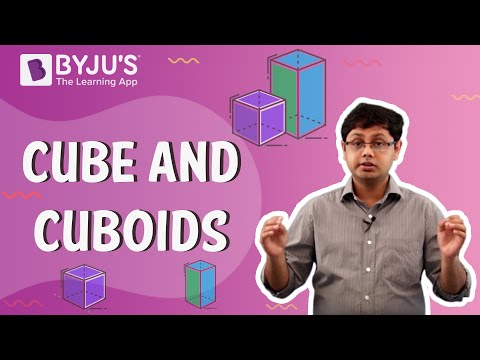To know more about Cubes and Cuboids, visit here.

### Right Circular Cylinder

A right circular cylinder is a closed solid that has two parallel circular bases connected by a curved surface in which the two bases are exactly over each other and the axis is at right angles to the base.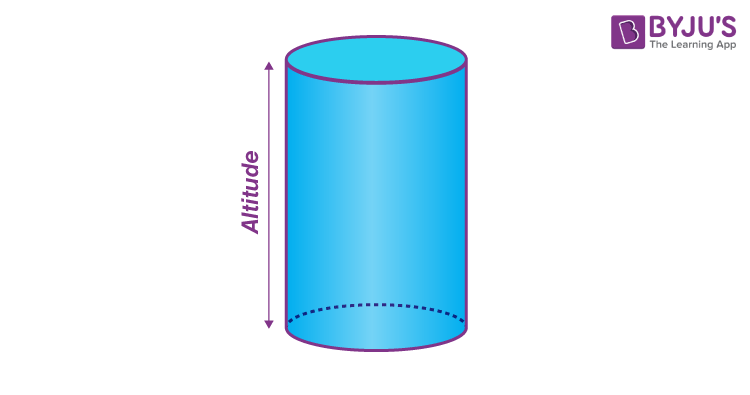### Curved Surface Area of a Right Circular Cylinder

Take a cylinder of base radius r and height h units. The curved surface of this cylinder, if opened along the diameter (d = 2r) of the circular base, will be transformed into a rectangle of length 2πr and height h units. Thus,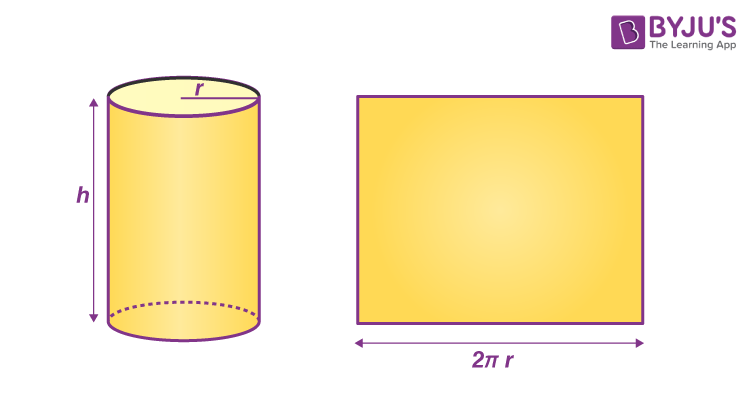Curved surface area(CSA) of a cylinder of base radius r and height h 2π × × h

### Total Surface Area of a Right Circular Cylinder

Total surface area(TSA) of a cylinder of base radius r and height h = 2π × × h + area of two circular bases
TSA 2π × × × πr2
TSA 2πr(r)

To know more about Right Circular Cylinder, visit here.

### Right Circular Cone

A right circular cone is a circular cone whose axis is perpendicular to its base.

### Relation between Slant Height and Height of a Right Circular Cone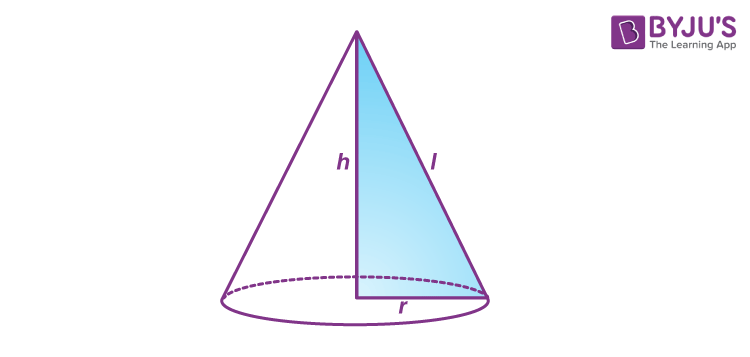The relationship between slant height(l) and height(h) of a right circular cone is:
lhr2    (Using Pythagoras Theorem)
Where r is the radius of the base of the cone.

### Curved Surface Area of a Right Circular Cone

Consider a right circular cone with slant length l and radius r.
If a perpendicular cut is made from a point on the circumference of the base to the vertex and the cone is opened up, a sector of a circle with radius l is produced, as shown in the figure below: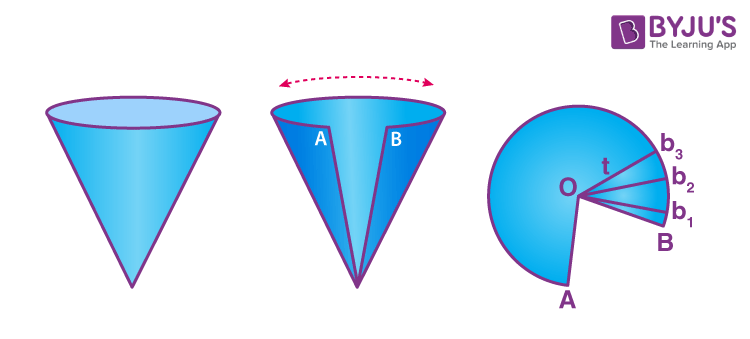Label A and B and corresponding b1, b2 …bn at equal intervals, with O as the common vertex. The Curved surface area(CSA) of the cone will be the sum of the areas of the small triangles: 1/2 × (bb2.……..bn× l
(bb2...bn) is also equal to the circumference of base 2πr
CSA of right circular cone (1/2) × (2πr× πrl          (On substituting the values)

### Total Surface Area of a Right Circular Cone

Total surface area(TSA) = Curved surface area(CSA) + area of base πrπrπr(r)

To know more about Right Circular Cone, visit here.

### Sphere

A sphere is a closed three-dimensional solid figure, where all the points on the surface of the sphere are equidistant from the common fixed point called “centre”. The equidistant is called the “radius”.

### Surface Area of a Sphere

The surface area of a sphere of radius r = 4 times the area of a circle of radius r =4×(πr2)
For a sphere Curved surface area (CSA) = Total Surface area(TSA) = 4πr2

To know more about Sphere, visit here.

### Surface Area Formulas for Class 9

 Shapes Surface Areas Cuboid 2(lb + bh + hl) Cube 6a2 Right Circular Cylinder 2πr(r + h) Right Circular Cone πr(l + r), ( l2 = h2 + r2 ) Sphere 4 π r2

### Volume of a Cuboid

The volume of a cuboid is the product of its dimensions.
Volume of a cuboid = lengt× breadt× height= lbh
Where l is the length of the cuboid, b is the breadth, and h is the height of the cuboid.

### Volume of a Cube

The volume of a cube = base are× height.
Since all dimensions are identical, the volume of the cube a3
Where a is the length of the edge of the cube.

### Volume of a Right Circular Cylinder

The volume of a right circular cylinder is equal to base area × its height.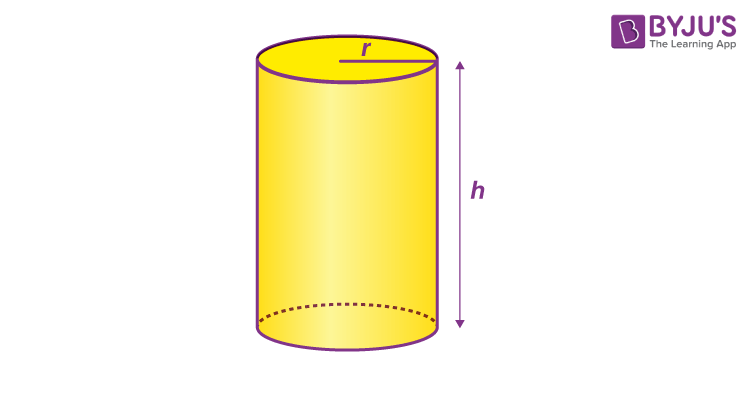The volume of a cylinder =πr2h
Where r is the radius of the base of the cylinder and h is the height of the cylinder.

### Volume of a Right Circular Cone

The volume of a Right circular cone is 1/3 times the volume of a cylinder with the same radius and height. In other words, three cones make one cylinder of the same height and base.
The volume of right circular cone (1/3)πr2h
Where is the radius of the base of the cone and is the height of the cone.

### Volume of a Sphere

The volume of a sphere of radius r =(4/3)πr3

To know more about Volume Formulas for Different Geometric Shapes, visit here.

### Volume Formulas for Class 9

 Shapes Volumes Cuboid length × breadth × height Cube a3 Right Circular Cylinder πr2h Right Circular Cone ⅓ πr2h Sphere 4/3 π r3

### Volume and Capacity

The volume of an object is the measure of the space it occupies, and the capacity of an object is the volume of substance its interior can accommodate. The unit of measurement of either volume or capacity is a cubic unit.

Test your knowledge on Surface Areas And Volumes Class 9

3. this is very useful for 9th notes

4. Good one notes

5. Thank you so much sir

6. REALLY IT HELPED ME A LOT BYJUS AND ALL TEACHERS HATS OFF

7. Thank you for helping me for the exams